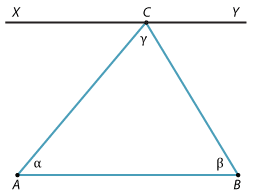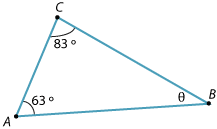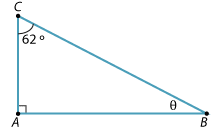Proving that the sum of the interior angles of a triangle is 180°

The statement of the result is called a theorem. This comes from a Greek word meaning 'a thing to be gazed upon' or 'a thing contemplated by the mind'. The word 'theatre' comes from the same Greek root.

Theorem

The sum of the angles of a triangle is 180°.Proof

Let ∆ABC be a triangle.

Let $$\angle$$BAC = α, $$\angle$$CBA = β and $$\angle$$BCA = γ.

We must prove that α + β + γ = 180°.

The line XY is drawn parallel to the line AB through the vertex C.

\begin{align}\text{Then}\ \angle XCA &= α\ (\text{alternate angles}\ XY || AB).\\\\ \angle YCB&= β\ (\text{alternate angles}\ XY||AB)\end{align}

Hence α + β + γ = 180° (straight angle at C).

Example 1

Find the value of θ in each of the following.

1.2.Solution

1. θ + 83° + 63° = 180° (angle sum of ∆ABC)
Hence θ = 34°.
2. θ + 90° + 62° = 180° (angle sum of ∆ABC)
Hence θ = 28°.